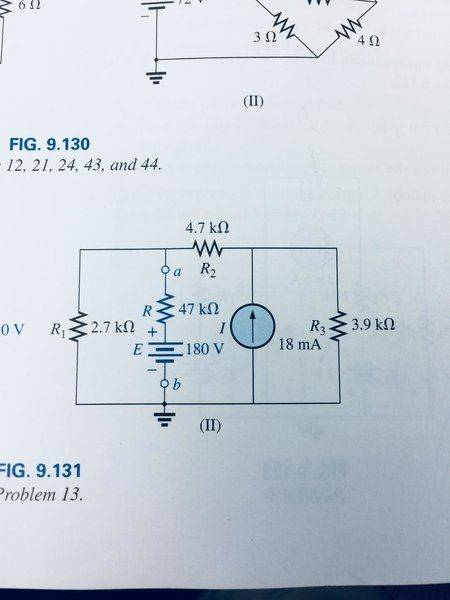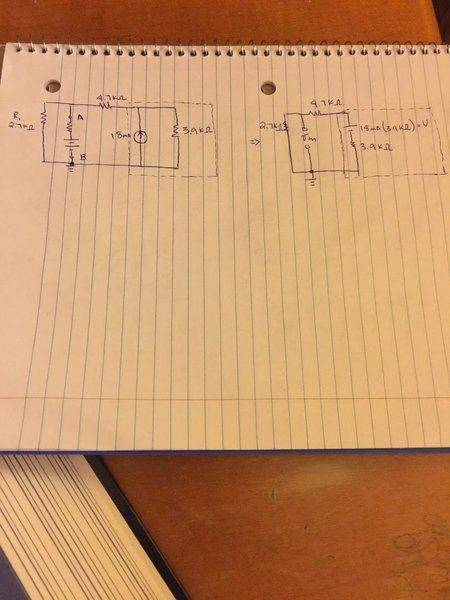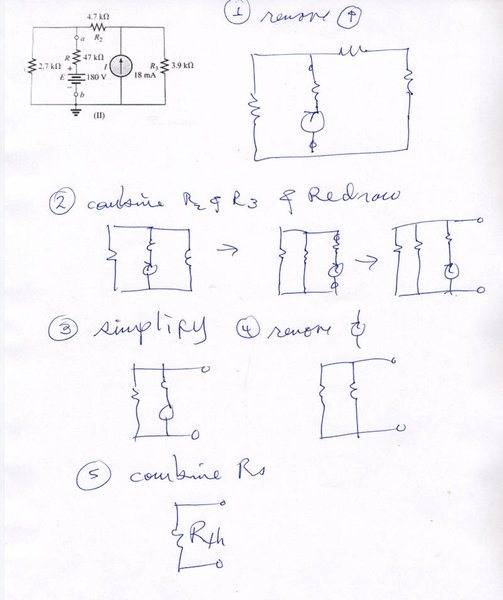# Find the Thevenin equivalent circuit

• Engineering
• icesalmon
On my calc (and I am a bit rusty on this) the voltage across R3 should be the voltage between terminals a and b, multiplied by (R1 + R2)/R1.2. You could also use ohms law and just multiply the voltage across R1 and R2 by the resistances.The resistance is indeed ##R_1||(R_2+R_3)##. Do you understand where you made the mistake in the previous post about the resistance?f

#### icesalmon

Homework Statement
Find the Thevenin equivalent circuit for the portions of the networks in the following circuit:## Homework Equations

Kirchoff's Voltage Law: ΣVdrops = ΣVgains
Kirchoff's Current Law: ΣIclosed loop = 0
Ohms Law: V = IR

## The Attempt at a Solution

Determination of the Thevenin Resistance is simple enough, Rth = 2.7 + ((4.7)(3.9)/(4.7+3.9)) = 2.05kΩ

The Thevenin Voltage is what's giving me trouble; if I measure the voltage across the terminals of the branch highlighted in blue how does the 3.9 kΩ resistor not experience any of this thevenin voltage?

#### Attachments

Last edited:
The Thevenin Voltage is what's giving me trouble; if I measure the voltage across the terminals of the branch highlighted in blue how does the 3.9 kΩ resistor not experience any of this thevenin voltage?

It does, doesn't it?

On my calc (and I am a bit rusty on this) the voltage across R3 should be the voltage between terminals a and b, multiplied by (R1 + R2)/R1.

Last edited:
It does, doesn't it?

On my calc (and I am a bit rusty on this) the voltage across R3 should be the voltage between terminals a and b, multiplied by (R1 + R2)/R1.
You're using a voltage divider, right? Okay isn't this saying that the thevenin voltage is only distributed over R1 and R2? I'm missing why it doesn't distribute over R3.

You're using a voltage divider, right? Okay isn't this saying that the thevenin voltage is only distributed over R1 and R2? I'm missing why it doesn't distribute over R3.
I'm afraid I'm not familiar with the term voltage divider.

Treating the alternate path between a and b (the one with the cells and the 47k-ohm resistor in) as open, there are two closed paths that are in parallel to the current source. One is R3 and the other is R1 in series with R2, with the terminal a between them. The voltage between the terminals is that across R1, so the voltage across the R1 and R2 series is that times (R1+R2)/R1. That has to be the same as the voltage across R3 because the R1,R2 series is in parallel with R3.

It may help to visualise if you redraw the circuit with the current source vertical in the middle, R3 vertical on the right and the R1,R2 series vertical on the left. Terminal b is at the bottom and terminal a is halfway up the left-hand path, between R1 and R2. Leave the cells and the 47k-ohm resistor undrawn. That makes clearer what the two paths parallel to the current source are.

The Thevenin Voltage is what's giving me trouble; if I measure the voltage across the terminals of the branch highlighted in blue how does the 3.9 kΩ resistor not experience any of this thevenin voltage?
What do you mean by the resistor not experiencing any of the voltage?

What do you mean by the resistor not experiencing any of the voltage?
The thevenin voltage isn't divided among R1, R2, and R3; only R1 and R2

Are you saying if you assume there's a voltage ##V## across the current source, then the Thevenin voltage is ##\frac{R_1}{R_1+R_2} V## and that expression doesn't seem to involve ##R_3##?If we transform the current source by using ohms law so we now have a circuit with three branches. Does the thevenin voltage get distributed over the branch with the voltage source and the 3.9kΩ resistor? ?

#### Attachments

Thevenin Resistance is simple enough, Rth = 2.7 + ((4.7)(3.9)/(4.7+3.9)) = 2.05kΩ
That's not correct. Take another look at it.

View attachment 228310
If we transform the current source by using ohms law so we now have a circuit with three branches. Does the thevenin voltage get distributed over the branch with the voltage source and the 3.9kΩ resistor? ?
I don't think you're using the word branch the way everybody else does. What do you mean the circuit has three branches?

I don't think you're using the word branch the way everybody else does. What do you mean the circuit has three branches?
I apologize, I can see that the network I used a source transformation on does not have that many branches.

That's not correct. Take another look at it.
Should it be 2.7 || ( 4.7 + 3.9) = 2.7 || 8.6 = (2.7*8.6)/(2.7 + 8.6) = 2.06kΩ = Rth?

Should it be 2.7 || ( 4.7 + 3.9) = 2.7 || 8.6 = (2.7*8.6)/(2.7 + 8.6) = 2.06kΩ = Rth?
That looks better.

Should it be 2.7 || ( 4.7 + 3.9) = 2.7 || 8.6 = (2.7*8.6)/(2.7 + 8.6) = 2.06kΩ = Rth?

The resistance is indeed ##R_1||(R_2+R_3)##. Do you understand where you made the mistake in the previous post about the resistance?
As for the thevenin equivalent voltage across ab, i will give you two ways to solve it and both are relatively simple with one being slightly simpler than the other.

1. Since you know about the voltage divider you must know about the current divider as well. No need to transform the current source, just use the current divider to find the current that circulates to the left (lets say it's ##I_L## going counterclockwise) and when you do that ##V_{th}=I_LR_1##. This one was the slightly harder approach.

2. Since you know the ##R_{th}## if you short-circuit the ab part of the wire and find the current going that part, multiply it with the ##R_{th}## you found and you will get the thevenin voltage across ab. ##I_NR_{th} = V_{th}##, ##I_N## is the Norton equivalent current through the ab branch.
Try it both ways.

The resistance is indeed ##R_1||(R_2+R_3)##. Do you understand where you made the mistake in the previous post about the resistance?
As for the thevenin equivalent voltage across ab, i will give you two ways to solve it and both are relatively simple with one being slightly simpler than the other.

1. Since you know about the voltage divider you must know about the current divider as well. No need to transform the current source, just use the current divider to find the current that circulates to the left (lets say it's ##I_L## going counterclockwise) and when you do that ##V_{th}=I_LR_1##. This one was the slightly harder approach.

2. Since you know the ##R_{th}## if you short-circuit the ab part of the wire and find the current going that part, multiply it with the ##R_{th}## you found and you will get the thevenin voltage across ab. ##I_NR_{th} = V_{th}##, ##I_N## is the Norton equivalent current through the ab branch.
Try it both ways.

Yes, the mistake was in thinking that all THREE resistors were in series, when in fact only the 4.7 and 2.7 kΩ resistors were in series and those were in parallel with the 3.9kΩ resistor, the current through ##R_{1}## and ##R_{2}## is not the same as that through ##R_{3}##, hence ##R_{12} = (R_{1}+ R_{2})## is in parallel with ##R_{3}##.

So ##V_{th}=I_{L}R_{1}## so I have ##I_L = 18mA*( (R_1 + R_2) / R_{123})## and I'm getting ##I_{L} = 11.8mA## which, at least is less than the 18 mA so it appears to be correct in value, but maybe I'm missing something here?

Last edited:
I'm afraid I'm not familiar with the term voltage divider.

Treating the alternate path between a and b (the one with the cells and the 47k-ohm resistor in) as open, there are two closed paths that are in parallel to the current source. One is R3 and the other is R1 in series with R2, with the terminal a between them. The voltage between the terminals is that across R1, so the voltage across the R1 and R2 series is that times (R1+R2)/R1. That has to be the same as the voltage across R3 because the R1,R2 series is in parallel with R3.

It may help to visualise if you redraw the circuit with the current source vertical in the middle, R3 vertical on the right and the R1,R2 series vertical on the left. Terminal b is at the bottom and terminal a is halfway up the left-hand path, between R1 and R2. Leave the cells and the 47k-ohm resistor undrawn. That makes clearer what the two paths parallel to the current source are.

when you say something like R3 vertical do you mean the portion of the circuit that current will travel through R3 in?

Last edited:
Yes, the mistake was in thinking that all THREE resistors were in series, when in fact only the 4.7 and 2.7 kΩ resistors were in series and those were in parallel with the 3.9kΩ resistor, the current through ##R_{1}## and ##R_{2}## is not the same as that through ##R_{3}##, hence ##R_{12} = (R_{1}+ R_{2})## is in parallel with ##R_{3}##.

So ##V_{th}=I_{L}R_{1}## so I have ##I_L = 18mA*( (R_1 + R_2) / R_{123})## and I'm getting ##I_{L} = 11.8mA## which, at least is less than the 18 mA so it appears to be correct in value, but maybe I'm missing something here?
The correct equation for the current divider is ##I_L=I\frac{R_3}{R_{123}}##. Then ##V_{th}=I_LR_1##.

The correct equation for the current divider is ##I_L=I\frac{R_3}{R_{123}}##. Then ##V_{th}=I_LR_1##.
##R_3## is on the right though, so wouldn't the current going to the left portion of the circuit pass through ##R_1## and ##R_2##?

##R_3## is on the right though, so wouldn't the current going to the left portion of the circuit pass through ##R_1## and ##R_2##?
http://www.basicsofelectricalengineering.com/2017/07/current-divider-rule.html. Check this out to see how the current divider is derived.
In simple terms, ##I_L=I\frac{\text{sum of opposite resistances}
}{\text{sum of all resistances}}##. In this case we see that in the left branch we have ##R_{12}## resistances and on the right (opposite side=whichever ones are not the branches that current we are looking for is going through) we have ##R_3## and you see the result i posted.

This is getting ridiculous. @icesalmon you will never get good at understanding these problems until you learn to redraw the circuit. Since you're new to it, I recommend step by step:As you do the reduction, you do the calc's along the way and then you don't end up w/ any long confusing formulae, just simple ones as you go. Such problems are often, as this one is, drawn in a way that confuses beginners. This is done deliberately so that you DO learn to redraw.

#### Attachments

This is getting ridiculous. @icesalmon you will never get good at understanding these problems until you learn to redraw the circuit. Since you're new to it, I recommend step by step:

View attachment 228358

As you do the reduction, you do the calc's along the way and then you don't end up w/ any long confusing formulae, just simple ones as you go. Such problems are often, as this one is, drawn in a way that confuses beginners. This is done deliberately so that you DO learn to redraw.

Not sure what is so "ridiculous" about trying to understand how norton current / thevenin voltages can be determined, and considering its one of the first topics covered in introductory circuit analysis i would feel my first pass through this material should be a careful and well thought out one, asking plenty of questions should not be something that is discouraged. I had no issues determining the thevenin resistance, i simply typed the wrong thing in my above post and was corrected on it, end of story. I have little experience with voltage and current dividers, so i conceptually thought about how current coming from the 18mA source would travel through the left and right sides of the circuit (through ##R_{12}## and ##R_3## seperately) instead of just memorizing formulae, i was directed to an article deriving the current and voltage division concept.

Not sure what is so "ridiculous" about trying to understand how norton current / thevenin voltages can be determined ...

The common problem with these explanations is that they are dealing with too much of the circuit at once to really be just the application of Thevenin/norton transformations. Thevenin/Norton is simply the change between a series voltage and resistance to a parallel current source and resistance. So, you would just work with a single source and a single resistance (or impedence for AC circuits) at each transformation; i.e. just a small piece of the whole circuit. Each transformation will convert (only) a single loop to a branch or a single branch to a loop. Then you can simplify the network by combining series/parallel elements of the same type, like two resistors into one equivalent resistor or two sources into one source. Then repeat the process: transform - simplify - transform - simplify, etc. until you have a very simple circuit to evaluate. At each step you are only working with two circuit elements.

... just a small piece of the whole circuit. Each transformation will convert (only) a single loop to a branch or a single branch to a loop. Then you can simplify the network by combining series/parallel elements of the same type, like two resistors into one equivalent resistor or two sources into one source. Then repeat the process: transform - simplify - transform - simplify, etc. until you have a very simple circuit to evaluate. At each step you are only working with two circuit elements.
Gee, why didn't I think of thatThis is getting ridiculous. @icesalmon you will never get good at understanding these problems until you learn to redraw the circuit. Since you're new to it, I recommend step by step:

View attachment 228358

As you do the reduction, you do the calc's along the way and then you don't end up w/ any long confusing formulae, just simple ones as you go. Such problems are often, as this one is, drawn in a way that confuses beginners. This is done deliberately so that you DO learn to redraw.

The problem wants the Thevenin equivalent at the a,b terminals with the 47 kΩ and 180 volt source not present, as in post #12.

The problem wants the Thevenin equivalent at the a,b terminals with the 47 kΩ and 180 volt source not present, as in post #12.
I completely disagree. The problem wants the Thevenin equivalent at the a,b terminals. Period.

As I said in post #20, these problems are often deliberately drawn in a way to force the use to learn how to redraw circuit.

I completely disagree. The problem wants the Thevenin equivalent at the a,b terminals. Period.

As I said in post #20, these problems are often deliberately drawn in a way to force the use to learn how to redraw circuit.

All the posters in posts #12, #13, #14 and myself think the problem wants Rth at terminals a,b without the 47k and 180V.

All the posters in posts #12, #13, #14 and myself think think the problem wants Rth at terminals a,b without the 47k and 180V.

I agree, the blue part should remain as it is and the rest of the circuit should be transformed. Perhaps the original problem isn't the transformation itself but rather something like finding the max power of the blue resistor which would explain why it is left out of the transformation but the OP wanted to pin-point the problem he was having.
Otherwise, it being of different color makes no sense.

... Otherwise, it being of different color makes no sense.
Good point. Guess I misread the problem.

I completely disagree. The problem wants the Thevenin equivalent at the a,b terminals. Period.
I'd really be surprised if your interpretation is correct. In my experience, when the circuit is drawn this way, whatever is between the two terminals is considered the load. Also, the fact that resistor R doesn't have a subscript like every other resistor in the problem suggests it's in a separate part of the circuit.

As I said in post #20, these problems are often deliberately drawn in a way to force the use to learn how to redraw circuit.
It's more likely that the authors drew it this way so students learn that they need to remove any load before finding the Norton or Thevenin equivalent.

There is probably a follow-on problem which wants the student to use the derived Thevenin equivalent to then determine the current through the (load?) resistor R when the 47k and 180V are then hooked back up.

The correct equation for the current divider is ##I_L=I\frac{R_3}{R_{123}}##. Then ##V_{th}=I_LR_1##.
I believe I have ##I_L = 18mA\frac{3.9kΩ}{2.06kΩ}## so ##I_L = 34mA##
I, then, have ##V_{th} = 34mA*(2.7kΩ) = 91.8V##

If ##R_{123} = R_{th} = 2.06kΩ## and ##I = 18mA## than I'm doing some arithmetic wrong here

The problem wants the Thevenin equivalent at the a,b terminals with the 47 kΩ and 180 volt source not present, as in post #12.
This is correct, ##V_{th}## is the voltage across that blue terminal, in this case ##R = R_L## and the 180V source need to be removed

It's more likely that the authors drew it this way so students learn that they need to remove any load before finding the Norton or Thevenin equivalent.
The outlined procedure in the text for thevenin's theorem explicitly states this must be done but on later problems the circuits are drawn more abstractly so the reader is almost forced to redraw the circuit in order to progress

I believe I have ##I_L = 18mA\frac{3.9kΩ}{2.06kΩ}## so ##I_L = 34mA##
I, then, have ##V_{th} = 34mA*(2.7kΩ) = 91.8V##